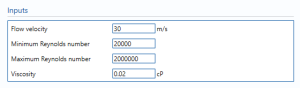# Flow Rate Gas Vortex

## InputsFlow velocity – the speed of the gas flowing through the gas vortex.

Minimum Reynolds number – The minimum Reynolds number attained.

Maximum Reynolds number – The maximum Reynolds number attained.

Note: Reynolds number is a dimensionless quantity that shows whether the flow is steady or turbulent.

Viscosity – the viscosity of the gas in the stream.

Note: Viscosity in gas is measure of resistance in flow.

## Uncertainty ParametersCalibration – Percentage uncertainty in calibration of the Gas Vortex.

Accuracy – The percentage uncertainty due to accuracy. Accuracy is the closeness of the measured value to the real value.

Drift – Additional uncertainty allowance due to meter drift. Normally left at zero, unless evidence is present to suggest a higher value.

Repeatability – Percentage uncertainty in the repeatability of the reading from the gas Vortex meter. Installation effects – The percentage uncertainty due to installation effects. Installation effects can be from several sources such as a distorted swirl in the profile of the flow due to the layout of the piping system.

Computation – Uncertainty due to computational errors in flow computer.

## Calculation ParametersReynold’s number – Reynolds number that is used in the calculation.

## Meter dataMeter factor – number of pulses generated by the vortex meter per unit volume or mass.

Internal pipe diameter – the internal diameter of the pipe.

Uncertainty in internal pipe diameter – the uncertainty of the measurement of the internal pipe diameter.

## Uncertainty Budget

### Volume flow rate

The uncertainty budget table shows a break down of the different components that contribute to the overall calculated uncertainty.The values input into the uncertainty budget are derived from the measured pressure and temperature that the transmitter specific values relates to its calibration and specification. These values are taken in as the expanded uncertainties and are divided by a coverage factor to gain the standard uncertainty.

The coverage factor is determined by the probability distribution that best suits that uncertainty component. The standard uncertainty is then multiplied by the sensitivity value then squared. This is done for each component that contributes to the overall uncertainty in volume flow rate. The Standard Uncertainty in the volume flow rate is the square root of the sum of each component variance as shown in the following equation:### Standard Volume flow rate

The uncertainty budget table shows a break down of the different components that contribute to the overall calculated uncertainty.The values input into the uncertainty budget are derived from the measured pressure and temperature that the transmitter specific values relates to its calibration and specification. These values are taken in as the expanded uncertainties and are divided by a coverage factor to gain the standard uncertainty.

The coverage factor is determined by the probability distribution that best suits that uncertainty component. The standard uncertainty is then multiplied by the sensitivity value then squared. This is done for each component that contributes to the overall uncertainty in standard volume flow rate. The Standard Uncertainty in the standard volume flow rate is the square root of the sum of each component variance as shown in the following equation:### Mass flow rate

The uncertainty budget table shows a break down of the different components that contribute to the overall calculated uncertainty.The values input into the uncertainty budget are derived from the measured pressure and temperature that the transmitter specific values relates to its calibration and specification. These values are taken in as the expanded uncertainties and are divided by a coverage factor to gain the standard uncertainty.

The coverage factor is determined by the probability distribution that best suits that uncertainty component. The standard uncertainty is then multiplied by the sensitivity value then squared. This is done for each component that contributes to the overall uncertainty in mass flow rate. The Standard Uncertainty in the mass flow rate is the square root of the sum of each component variance as shown in the following equation:### Energy flow rate

The uncertainty budget table shows a break down of the different components that contribute to the overall calculated uncertainty.The values input into the uncertainty budget are derived from the measured pressure and temperature that the transmitter specific values relates to its calibration and specification. These values are taken in as the expanded uncertainties and are divided by a coverage factor to gain the standard uncertainty.

The coverage factor is determined by the probability distribution that best suits that uncertainty component. The standard uncertainty is then multiplied by the sensitivity value then squared. This is done for each component that contributes to the overall uncertainty in energy flow rate. The Standard Uncertainty in the energy flow rate is the square root of the sum of each component variance as shown in the following equation:### Calculated UncertaintyThe Expanded Uncertainty is the Standard Uncertainty multiplied by the coverage factor (k). The coverage factor is defaulted to k = 2 (equivalent to a confidence level of approximately 95%).

The Relative Uncertainty is the Expanded Uncertainty divided by the volume flow rate, standard volume flow rate, mass flow rate, and energy flow rate depending on the parameter.

Back to Uncertainty Modules